# A New Approach to ICSE Physics Part 1 Class 9 Solutions Pressure in Fluids

## A New Approach to ICSE Physics Part 1 Class 9 Solutions Pressure in Fluids

These Solutions are part of A New Approach to ICSE Physics Part 1 Class 9 Solutions. Here we have given A New Approach to ICSE Physics Part 1 Class 9 Solutions Pressure in Fluids.

Practice Problems 1.
Question 1.
Calculate pressure exerted by 0.8 m vertical length of alcohol of density 0.80 gcnr5 in SI units.
[Take g = 10 ms-2].
Vertical length of alcohol column = h = 0.8 m
Density of alcohol = ρ = 0.80 g cm -3
$$\rho=\frac{0.80 \times 10^{6}}{10^{3}} \mathrm{kgm}^{-3}$$
ρ = 0.80 x 1000 kgm-3 .
ρ = 800 kg m-3
Pressure = P = ?
p= hpg
P = 0.8 x 800 x 10
= 6400 Pa

Question 2.
What is the pressure exerted by 75 cm vertical column of mercury of density 13600 kgm-3 in SI units.
[Take g = 9.8 ms-2].
Vertical length of mercury column = h = 75 cm = 0.75 m
Density of mercury = p = 13600 kg m-3
Acceleration due to gravity = g = 9.8 ms-2
Pressure = P = ?
p= hpg
P = 0.75 x 13600×9.8
P = 99960 Pa

Practice Problems 2.
Question 1.
66640 Pa pressure is exerted by 0.50 m vertical column of a liquid. If g = 9.8 Nkg-1, calculate density of the liquid.
Pressure + P = 66640 Pa .
Vertical length of liquid column = h = 0.50 m
Acceleration due to gravity -g = 9.8 ms-2
$$\rho=\frac{66640}{0.50 \times 9.8}$$
ρ = 13600 kg m-3

Question 2.
What vertical height of water will exert pressure of 333200 Pa? Density of water is 1000 kgnr3 and g = 9.8 ms-2.
Vertical height of water = h = ?
Pressure due to water column = P = 333200 Pa Acceleration due to gravity = g = 9.8 ms-2Question 3.
Pressure at bottom of sea at some particular place is 8968960 Pa. If density of sea water is 1040 kgm3 calculate the depth of sea. Take g = 9.8 ms-2. Neglect the pressure of the atmosphere.
Pressure at the bottom of the sea = P = 8968960 Pa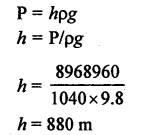Practice Problems 3.

Question 1.
Atmospheric pressure at sea level is 76 cm of mercury. Calculate the vertical height of air column exerting the above pressure. Assume the density of air 1.29 kgm-3 and that of mercury is 13600 kgm-3. Why the height calculated by you is far less than actual height of atmosphere?Question 2.
Calculate the equivalent height of mercury, which will exert as much pressure as 960 m of sea water of density 1040 kgm-3. Density of mercury is 13600 kgm-3.Practice Problems 4.
Question 1.
The pressure of water on the ground floor, in a water pipe is 150000 Pa, whereas pressure on the fourth floor is 30000 Pa. Calculate height of fourth floor. Take g = 10 ms-2.
Pressure of water at fourth floor = P2 = 3000 Pa Let h be the height of fourth floor
Difference in pressure of water at ground floor and fourth floor
= P1 – P2 = 150000 – 30000
= 120000 Pa
Pressure of water due to height (h) = hρg
=> P1 – P2 = h p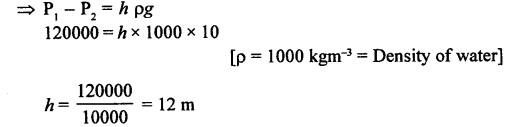Question 2.

The pressure of water on ground floor is 160000 Pa. Calculate the pressure at the fifth floor, at a height of 15 m.
Pressure of water at ground floor = P, = 160000 Pa
Pressure of water at fifth floor = P2 = ?
Height of fifth floor = h = 15 m
Density of water = ρ = 1000 kgm-3
Difference in pressure of water at ground and fifth floor
= P1 – P2
Pressure of water due to height (h) = hρg
= P1 – P2 = hρg
160000 -P2= 15 x 1000 x 10
P2= 160000-150000
P2 = 10000 Pa

Practice Problems 5.
Question 1.
(a)
The area of cross-sections of the pump plunger and press plunger of a hydraulic press are 0.02 m2 and 8 m2 respectively. If the hydraulic press overcomes a load of 800 kgf, calculate the force acting on pump plunger.
(b) If the mechanical advantage of the handle of pump plunger is 8, calculate the force applied at the end of the handle of pump plunger.
(a) Load on the press plunger = L = 800 kgf
Let the effort acting on the pump plunger = E(b)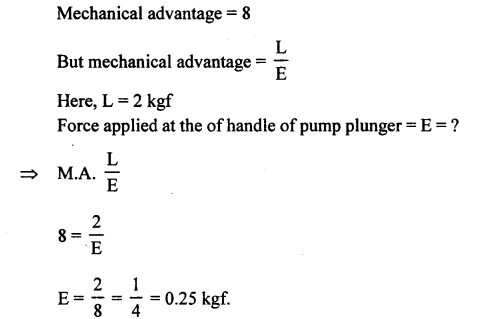Question 2.
The radii of the press plunger and pump plunger are in ratio of 50 : 4. If an effort of 20 kgf acts on the pump plunger, calculate the maximum effort which the press plunger can over come.
Effort acting on the pump plunger – E = 20 kgf
Load acting on the press plunger = L = ?
So let radius of pump plunger = 5 Ox = R and radius of press plunger = Ax = r

$$\text { Now, } \frac{L}{E}=\frac{\pi R^{2}}{\pi r^{2}}$$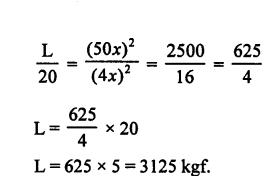QUESTIONS BASED ON ICSE EXAMINATION
(A) Objective Questionsion

Multiple Choice Questions.
Select the correct option.

1. Unit of thrust in SI system is
(a) dynes
(b) joule
(c) N/m2
(d) newton

2. The unit Nm2 is the unit of
(a) force
(b) pressure
(c) thrust
(d) momentum

3. One Pascal is equal to:
(a) Nm2
(b) Nm-2
(c) Nm2
(d) Nm-1

4. Thrust acting perpendicularly on the unit surface area is called :
(a) pressure
(b) moment of force
(c) down thrust
(d) none of these

5. Pressure applied in liquids is transmitted with undiminished force:

(a) in downward direction
(b) upward direction only
(c) sides of containing vessel
(d) in all directions

6. As we move upwards, the atmospheric pressure :
(a) increases
(b) decreases
(c) remains same
(d) cannot be said

7. A dam for water reservoir is built thicker at the bottom than at the top because :
(a) pressure of water is very large at the bottom due to its large depth

(b) water is likely to have more density at the bottom due to its large depth
(c) quantity of water at the bottom is large
(d) variation in value of ‘g’

8. The pressure exerted by 50 kg (g = 10 m/s2) on an area of cross section of 2 m2 is :
(a) 50 Pa
(b) 200 Pa
(c) 250 Pa
(d) 1000 Pa
Ans. (c) 250 Pa
Explanation : m = 50 kg; g = 10 ms-2
Area of cross-section = A = 2m2
Pressure $$P=\frac{F}{A}=\frac{m g}{A}=\frac{50 \times 10}{2}$$=250Pa

9. Pressure at a point inside a liquid does not depend on :
(a) The depth of the point below the surface of the liquid
(b) The nature of the liquid
(c) The acceleration due to gravity at that point
(d) The shape of the containing vessel

10. The atmospheric pressure on earth’s surface is approximately
(a) 105 Pa

(b) 104 Pa
(c) 9.6 x 104 N/m2
(d) 10-4 Pa

(B) Subjective Questions

Question 1.
State three factors on which the pressure at a point in a liquid depends.
Factors on which the pressure at a point in a liquid depends are:

1. Pressure in a liquid is directly proportional to its height or depth.
2. Pressure in a liquid is directly proportional to its density.
3. Pressure in a liquid is directly proportional to the acceleration due to gravity.
4. Pressure in a liquid is independent of the area of cross­section.

Question 2.
The normal pressure of air is 76 cm of mercury. Calculate the pressure in SI units.
[Density of mercury = 13600 kg/m3 and g = 10 m/s2]
Height of mercury column = h = 76 cm
h = 0.76 m
Density of mercury =ρ = 13600 kg/m3
Acceleration due to gravity = g = 10 m/s2
Pressure = P = ?
P = hρg
P = 0.76 x 13600 x 10 = 103360 N/m2

Question 3.
At a given place, a barometer records 70 cm of Hg. If the mercury in barometer is replaced by water, what would be resulting reading?  (Density of Hg = 13600 kg/m3; Density of water = 1000 kg/m3)
Height of mercury column = h = 70 cm = 0.70 m
Density of mercury = ρ= 13600 kg/m3
Acceleration due to gravity = g = 10 m/s2
Pressure = hρg
For water:
Height of water column = h’ = ?
Pressure due to water column = P’Question 4.
The base of cylindrical vessel measures 300 cm2. Water is poured into it upto a depth of 6 cm. Calculate the pressure of water on the base in vessel.
Area of base of cylinder = A = 300 cm2
A = 300 x 10 m2
A = 3 x 10m2
Height (or depth) of water column = h = 6 cm
$$h=\frac{6}{100} \mathrm{m}$$
Density of water = ρ = 1000 kg/m3
Acceleration due to gravity = g = 10 m/s2
Pressure at the base in vessel = P = hρg
ρ = $$\frac{6}{100}$$ x 1000 x 10
P = 600Pa

Question 5.
The pressure in water pipe on the ground floor of a building is 40000 pascals, whereas on the first floor it’s 10000 pascals. Find the height of first floor. (Acceleration due to gravity g = 10 ms-2)
Ans.
Pressure on the ground floor of a building = P1 = 40000 Pa
Pressure on the first floor of a building = P2 = 10000 Pa
ρ = 1000 kg/m3 = density of water
Acceleration due to gravity = g = 10 m/s2
Difference in pressure = P, – P2 = 40000 – 10000 = 30000 Pa
Let h = height of first floor
Pressure of water due to height (h) = hρg
30000 = h x 1000 x 10
$$h=\frac{30000}{10000}=3 \mathrm{m}$$

Question 6.
(a) Define SI unit of pressure.
(b) The atmospheric pressure at a place is 650 mm of Hg. Calculate this pressure in Pascals (Pa).
(a) SI unit of pressure is pascal (Pa) or Nm-2
One Pascal: When a force of one newton acts normally on an area of one square metre (1 m2) then pressure acting on the surface acting on the surface is called one Pascal.
(b) Height of mercury column = h = 650 mm
h = 65 cm = 0.65 m
Density of mercury = ρ= 13600 kg/m3
Acceleration due to gravity = g = 10 ms-2
Pressure (P) = 650 mm of Hg = hρg
= 0.65 x 13600=10
= 88400 Pa

Question 7.
Pressure in a water pipe on the ground floor of a building is 100,000 Pa. Calculate the pressure in water pipe on first floor at a height of 3 m. [Density of water = 1000 kgm-3 ; g = 10 ms-2]
Pressure of water at ground floor = P1 = 1,00,000 Pa
Pressure of water at first floor = P2 = ?
Height of first floor = h = 3 m
Density of water = ρ= 1000 kgm-3
Acceleration due to gravity = g = 10 ms-2
Difference in pressure of water at-ground floor an first floor
= P1 -P2
Pressure of water due to height (h) = hρg
=> P1– P2 = hρg
1,00,000 – P2 = 3 x 1000 x 10                                        –
P2= 1,00,000-30000
P2= 70000 Pa
∴ Pressure of water in pipe at first floor of a building is 70000 Pa.

Question 8.
P is the pressure at some point in a liquid. State whether pressure P is a scalar or vector quantity.
Pressure exerted on an enclosed fluid gets transmitted equally and undiminishingly in all directions. So no particular direction is associated with pressure.
That is why Pressure is a scalar quantity.

Question 9.
A beaker contains a liquid of density ‘ρ’ upto height ‘h’such that ‘PA’ is atmospheric pressure and ‘g’ is acceleration due to gravity. Answer the following questions :
(a) What is the pressure on the free surface of liquid?
(b) What is the pressure on the base of beaker?
(c) What is the lateral pressure at the base on the inner walls of beaker?
(a) Pressure on the free surface of liquid is equal to the atmospheric pressure (Pa).
(b) Consider a liquid contained in a beaker, such that ‘ p’ is the density of liquid.
Consider a point B at the base of liquid and the liquid column of area of cross-section ‘a’ around it, such that ‘h’is the
height of the liquid column as shown in the figure.(c) Also lateral pressure at the base on the inner walls of beaker = Pa + hρg

Question 10.
State the law of transmission of pressure in liquids.
Ans.
Pascal’s law : “The pressure applied on the surface of a confined liquid is transmitted equally and undiminished in all directions throughout the liquid.”

Question 11.
Calculate the hydrostatic pressure exerted by water at the bottom of a beaker. Take the depth of water as 40 cm, the density of water 1000 kgm-3 and g = 9.8 ms-2.
Ans.
Pressure at the bottom of beaker = P = ?
Height (or depth) of water in beaker = h = 40 cm
h= 0.4m
Density of water =ρ
= 1000 kgm-3 Acceleration due to gravity = g = 9.8 ms-2
P = hρg
P = 0.4 x 1000 x9.8
= 3920 Pa

Question 12.
State Pascal’s law of transmission of pressure in a liquid.
Pascal’s law : “The pressure applied on the surface of a confined liquid is transmitted equally and undiminished in all directions throughout the liquid.”

Question 13.
State briefly, how and why the atmospheric pressure of a place varies with the altitude. Draw an approximate graph to illustrate this variation.

1. We know atmospheric pressure = height of air column x density of air x acceleration due to gravity; P = hρg So, as we go up i.e. at higher altitudes, height of air column and hence atmospheric pressure decreases.
2. Also with the increase in altitude, density of air decreases and hence atmospheric pressure decreases.
If we take average density of air as 1.29 kgm-3 and the density of mercury as 13 600 kgm-3
we can find the height
Column which will exert as much pressure as*’ is exerted by 1 cm or (0.01 m) column of mercury as
Height of air column x density of air = height of mercury column x density of mercury height of air column x 1.29 kg/m3
= 0.01 m x 13600 kg/m3

∴ Height of air column = $$\frac{136}{1.29} \mathrm{m}$$=105m(approx)

Thus, 105 m of air column, on the average, will exert as much pressure as 1 cm column of mercury. Further,1 cm of mercury column exerts pressure = 105 m of air column 76 cm of mercury column exerts pressure = 105 x 76 m = 7980 m = 8 km (approx).
Thus, 8 km of air column will exert as much pressure as 76 cm of mercury column. However, it does not mean that atmosphere extends to only 8 km. As it is pointed out earlier, the density of atmosphere also changes with height. Thus, a fall of one cm in pressure does not mean that we have covered a vertical height of 105 m. On higher altitudes the vertical height of air is far in excess of 105 m, because of low density of air. A graph showing fall in pressure with height is shown in the figure.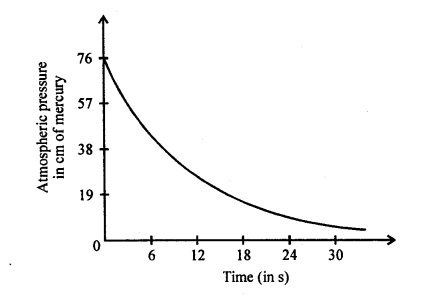Question 14.
The blood pressure reading of a patient is recorded 160/ 100. Express the lower pressure in SI units.
[Take density of mercury as 13.6 x 103 kgm-3 and the value of ‘g’ as 10 ms-2]
Lower pressure of the patient = 100 mm of Hg column
Height of mercury column = h = 100 mm = 10 cm h = 0.1 m
Density of mercury = ρ=13.6x 103 kgm-3
Acceleration due to gravity = g = 10 ms-2
So lower pressure of patient = P = hρg
P = 0.1 x 13.6 x 103 x 10
P = 13600 Pa

Question 15.
State two advantages of aneroid barometer.

1. It is compact, portable and hence can be carried anywhere.
2. It does not contain any liquid and there is no chance of spilling over of liquid as in mercury barometer.

Question 16.
Explain, why a gas bubble released at the bottom of a lake grows in size as it rises to the surface of the lake.